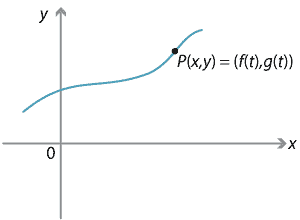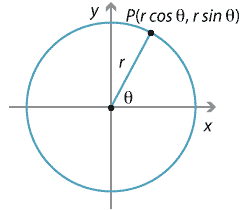### Parametric equations of a parabola

Since every parabola is congruent to $$x^2=4ay$$, for some choice of $$a > 0$$, we can study the geometry of every parabola by confining ourselves to this one! This shows the power of both coordinate geometry and transformations.

Imagine a particle moving in the plane along a curve $$C$$ as shown.Detailed description of diagram

The $$x$$- and $$y$$-coordinates of the particle can be thought of as functions of a new variable $$t$$, and so we can write $$x=f(t)$$, $$y=g(t)$$, where $$f,g$$ are functions of $$t$$. In some physical problems $$t$$ is thought of as time.

The equations

\begin{align*} x &= f(t)\\ y &= g(t) \end{align*}

are called the parametric equations of the point $$P(x,y)$$ and the variable $$t$$ is called a parameter.

It is often very useful to take a cartesian equation $$y=F(x)$$ and introduce a parameter $$t$$ so that the $$x$$- and $$y$$-coordinates of any point on the curve can be expressed in terms of this parameter.

Here is a very simple example of a parametrisation. Suppose we begin with the line whose equation is $$y=3x-4$$. We can introduce a new variable $$t$$ and write $$x=t$$. Then we have $$y=3t-4$$. Thus we can rewrite the equation $$y=3x-4$$ in parametric form as

\begin{align*} x &= t\\ y &= 3t-4. \end{align*}
##### Exercise 14

Eliminate the parameter $$t$$ from the equations

\begin{align*} x &= 2t-3\\ y &= 5-3t \end{align*}

and describe the resulting function.

Another common parametrisation is that used for a circle. The cartesian equation of a circle of radius $$r$$ centred at the origin is $$x^2+y^2 = r^2$$. We can put $$x = r\cos\theta$$, $$y = r\sin\theta$$, and we see that

$x^2+y^2 = r^2\cos^2\theta + r^2\sin^2\theta = r^2(\cos^2\theta + \sin^2\theta) = r^2,$

so these satisfy the original equation. The angle $$\theta$$ here is the parameter and measures the angle between the positive $$x$$-axis and a radial line to the circle.Detailed description of diagram

Thus we can describe the circle parametrically by

\begin{align*} x &= r\cos\theta\\ y &= r\sin\theta, \end{align*}

where $$0\leq \theta < 2\pi$$.

The parabola $$x^2=4ay$$, where $$a > 0$$, may also be parametrised and there are many ways to do this. We will choose the parametrisation

\begin{align*} x &= 2at\\ y &= at^2. \end{align*}

The parameter in this case is $$t$$.

This is the standard parametrisation for the parabola $$x^2=4ay$$ and the reason for this parametrisation will become apparent later in this module.

You should check that this parametrisation actually satisfies the original equation.

#### Example

Express the parabola $$x^2= 12y$$ parametrically.

#### Solution

In this case we see that $$a=3$$ and so the general point on the parabola can be expressed parametrically as

\begin{align*} x &= 6t\\ y &= 3t^2. \end{align*}

Next page - Content - Chords, tangents and normals